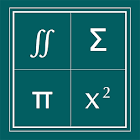Math Formulas

All Android applications categories

All Android games categories# Math Formulas

by: 5.2K 8.4

8.4 Users
rating

## Screenshots

Description

Use Menu button for fast collapse of expanded lists, swipe horizontally to view images and formulas completely

The App covers following topics

1) History
2) Numbers
3) Algebra
4) Geometry
5) Analytical Geometry
6) Trigonometry
7) Differential Calculus
8) Integrals Indefnite
9) Integrals Defnite
10) Sets
11) Statistics
12) Probability
13) Matrices
14) Vectors
15) Series
16) Boolean Algebra

The app is continuously updated with latest details and added with new topics frequently.

Tags: lications stylus math formulas , math all formula , math formulas , maths formula wallpaper 11 class , statistics formulas wallpaper

## Users review

from 5.165 reviews

"Great"

8.4